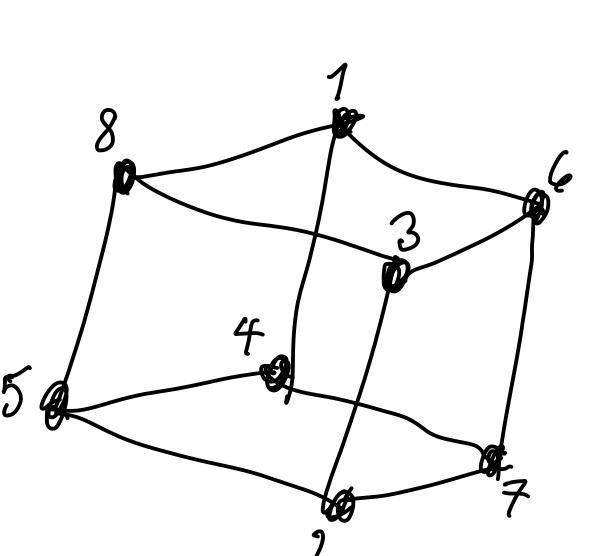## New blog post

Visible to anyone in the world

What interesting facts do you notice about how the corners of this cube are numbered?Share post

### New comment

The neighbouring numbers are opposite corners.

And 1-4 and 5-8 are opposite too.

### New comment

Total of corners for each and every face = 18

Jan

### New comment

Pairs of opposite edges?

### New comment

That's what I said. Is that it?

### New comment

Pairs of opposite edges like 14 and 35 have the same sum, not sure if that is to say?

If we join up each of the even numbers to all the others, so we have six joining lines, what do they form the ages of?

### New comment

From very different perspective,

Let point 5 be (-1,-1,-1), point 2 be (+1,-1,-1), point 7 be (+1,+1,-1), point 4 be (-1,+1,-1),

point 8 be (-1,-1,+1), point 3 be (+1,-1,+1), point 6 be (+1,+1,+1), point 1 be (-1,+1,+1)

and,

N5=(1-x)(1-y)(1-z)/8, N2=(1+x)(1-y)(1-z)/8, N7=(1+x)(1+y)(1-z)/8, N7=(1-x)(1+y)(1-z)/8,

N8=(1-x)(1-y)(1+z)/8, N3=(1+x)(1-y)(1+z)/8, N6=(1+x)(1+y)(1+z)/8, N1=(1-x)(1+y)(1+z)/8

where, -1<=x<=1, -1<=y<=1, -1<=z<=1

So, if the temperatures of these points are t5,t2,t7,t4,t8,t3,t6,t1,

the temperature at (x,y,z) is

t=N5*t5+N2*t2+N7*t7+N4*t4+N8*t8+N3*t3+N6*t6+N1*t1

### New comment

Joining evens forms a tetrahedron.

Joining odds forms another tetrahedron.

Jan

### New comment

Hi, Richard, Jan, everyone.

My idea may be too far from this discussion, very sorry.

### New comment

Nothing is too far, we have a structure with features that we can see in an elementary way but also may have some deeper maths underlying them.

Thanks, Richard.